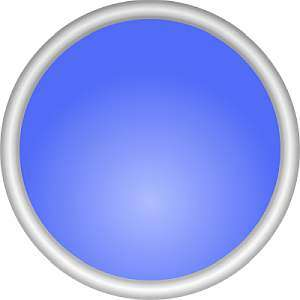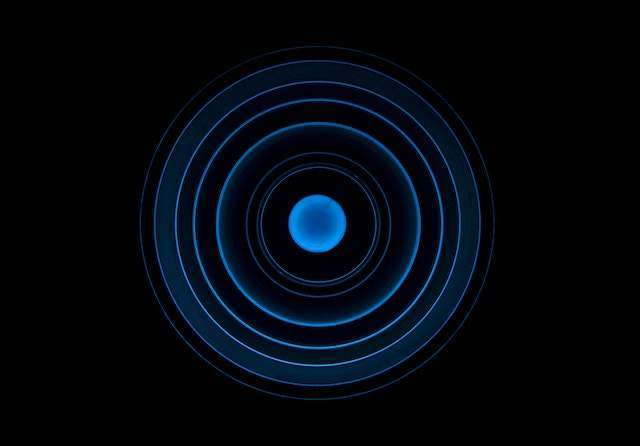# Circumference Formula Calculator

Instructions: Use this circumference formula calculator to compute the value of the circumference for a circle with a given radius r, provided in the box below.Enter the radius $$r$$ of the circle. Must be positive (Ex: 2, or 1/3, etc.)

## More about the circumference of a circle

This calculator will allow you compute the circumference of a circle for a given radius that is provided. So then, you need to provide a valid expression for the radius. It can be any valid numeric expression, such as '2' or '1/3'. The only restriction is that it must positive.

Once you provide a valid radius, the circumference formula will be used, with all steps shown.

The circumference is a measure of the length obtained if we would get a circle and we could "straighten it", almost as if the circle is made up of a thin noddle, and we could cut it, and actually measure the length of the noodle.## How to Calculate the Circumference of a Circle?

The idea of the circumference of a circle as a length is a geometrically clear one, but one that is not that easy to translate in to an actually measurement. It took mathematicians centuries to actually come up with the formula for the circumference of a circle:

$\displaystyle C = 2 \pi r$

Simple indeed, but very conceptual. Mathematicians were looking for something simple, but they were looking for something more "square". This enigmatic constant $$\pi$$ was the necessary conceptual step to fundamentally differentiate squares and circles.

## How to compute the circumference of a circle with radius

• Step 1: Identify the radius of the circle that is provided. If it is negative, then you cannot proceed
• Step 2: The the equation $$C = 2 \pi r$$, and plug in the known value of r
• Step 3: If the actual circumference depends on $$\pi$$ you can leave it in terms of $$\pi$$, or use a calculator to evaluate its numeric value
• Step 4: If r is given with length units, use the same length unit for the final result of the circumference

The process to find the circumference of a circle and the calculation of the circumference are trivial by just using the formula, plugging the value of r in it.

## What is the circumference for a radius 1?

A circle with radius 1 is called a unit circle. For that radius, the circumference is $$C = 2 \pi \cdot 1 = 2\pi$$.

Unit circles are broadly used in applications, but they are specially useful in the calculation of trigonometric amounts, which leads to crucial associations when putting them together with Pythagoras Theorem for example.

## Question: What a circumference is?

Though the calculation is trivial, you may still be thinking what an actual circumference is, and what it represents. And it makes sense, because it took mathematicians from the ancient Greeks a lot of thought to come up with an answer to it.

The circumference is the length of the circle, or at least that is how it is defined. Now, although the idea of the existence of a well defined length of a circle is easily accepted, it is a conceptual challenge to get into an agreement on how "length" is defined.

## Diameter to circumference calculator

• Step 1: If instead of a radius, you are provided with the diameter d, identify it and its possible length unit. It needs to be positive, otherwise you cannot proceed
• Step 2: Since we know that d = 2r, we simply compute the radius r by dividing the diameter by 2
• Step 3: Then, we use the usual equation $$C = 2 \pi r$$, and plug in the value we calculated for r

Other way to see it is to directly calculate the circumference from the diameter using the formula $$C = \pi d$$.

## Why would care about the circumference formula if I can use a calculator

Fair point. Though in this particular case it is crucial to understand where the circumference formula comes from. It is a very simple formula, but it also has a deep meaning. You can use a circumference calculator for sure, especially if it shows you the steps like this one.

There are multiple applications of the circumference of a circle, and more importantly, it gives rise to a lot of other concepts.### Example: Calculating the circumference

Use the circumference formula to find the circumference of a circle with radius $$r =\frac{3}{4}$$.

Solution: We need to find the circumference $$C$$ of the circle, and from the information provided, we know that the radius of the circle is $$r = \frac{3}{4}$$.

Now, the formula for the circumference is $$C = 2\pi r$$, and therefore, all we need to do is to plug into this formula the known value of the known radius $$r = \frac{3}{4}$$. The following is obtained:

$\begin{array}{ccl} C & = & \displaystyle 2 \pi r \\\\ \\\\ & = & \displaystyle 2 \pi \cdot \frac{3}{4} \\\\ \\\\ & = & \displaystyle \frac{3}{2}\pi{} \end{array}$

This concludes the calculation. We have found that the circumference of the circle is therefore $$\displaystyle C = \frac{3}{2}\pi{}$$.

### Example: Another calculation of the circumference

Now, assume that the diameter of the circle is d = 2. Calculate its circumference.

Solution: We need to find the circumference $$C$$ of the circle, and the diameter is known to be d = 2. Since the diameter must be equal to twice its radius, we conclude that the radius of the circle is $$r = 1$$.

Hence, using the formula for the circumference:

$C = \displaystyle 2 \pi r = 2 \pi \cdot 1 = 2\pi$

so then, the circumference is $$\displaystyle C = 2\pi{}$$.

### Example: Another example

Is it possible to find the circumference of a circle for a radius r = -3?

Solution: No. In order to find a valid circumference, the radius needs to be positive.

## Other useful related calculators

There are plenty of other things you would like to do with circles. For example, you may want to compute the area of a circle, or find the equation of a circle. Notice that the calculation of the perimeter only requires the radius, not the whole equation of the circle.

Some times, you will have the equation of the circle in general form, which may take some manipulation of the circle formula to find the radius and computer its circumference.